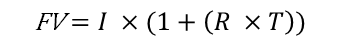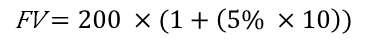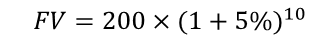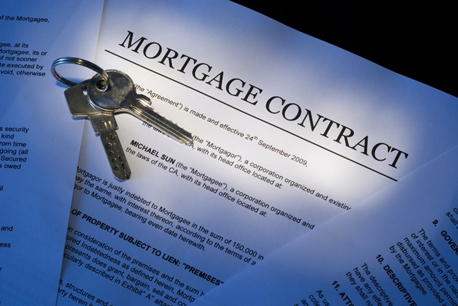# Future Value (FV)

• Updated on

## What is Future Value (FV)?

Future value is based on the concept of time value of money. According to time value of money, a dollar today is worth more than a dollar at a later point of time because factors like inflation and opportunity cost impact the value of money.

For instance, the purchasing power of US\$10 will not be the same ten years later as compared to what it can buy today because inflation will drive up the prices of products over the period. Similarly, from an investment perspective, there is always an opportunity cost of returns lost. A dollar today can be invested to earn additional returns at the end of 10 years, so the amount received at a later point must reflect the potential returns lost.

Summary
• The Future Value of an asset is essentially what it is predicted to be worth in the future.
• Future value helps investors and investment professionals estimate the potential returns from an investment at the end of the investment timeframe.
• The complexities associated with the future value depend on the nature of the asset, economic conditions, business cycles or any other unforeseeable factors.

## What is the importance of the concept of future value?

Future value is one of the primary and essential concepts in the world of finance. It helps investors and investment professionals estimate the potential returns from an investment at the end of the investment timeframe. A dollar invested in multiple assets over the same time could potentially give different returns at the end depending on the growth rate.

Hence, it is advisable to estimate the future values and compare them with the anticipated needs while investing. However, it must be noted that external factors like inflation, GDP growth, business cycles, other unforeseeable factors could deviate the actual values at the end from the estimated future values.Credit Cards use the FV concept, © Nebojsa78 | Megapixl.com

Using future value formulae, one can estimate the value of an asset at a particular point of time in the future, assuming all factors remain constant. An investor might have the option to retain his cash or to invest in multiple avenues like bonds, shares, commodities etc. Each of these options might have a plethora of options that yield different returns. For instance, fixed-rate bonds will have fixed returns over the period. On the other hand, shares will have varying returns over the period. Future value allows the investor to estimate all these options at a future date which makes it comparable.

How can future value be calculated?

The basic premise of Future Value is built on simple interest rate and compound interest rate. We will go through an example of each to understand the concept in detail.

• Calculating Future Value using simple interest rates

In a simple interest rate investment, the asset earns a specified return on the principal, or the initial investment, at the specified intervals. The critical differentiator in a simple interest investment is that the return is earned only on the principal and not on the incremental interest earned. A simple savings bank account is a classic example of a simple interest rate asset.

The future value of a simple interest rate asset can be calculated using the following equation-Where

FV = Future Value

I = Initial investment

R = Rate of interest

T = Time period

For example, let's assume a savings bank account with an initial investment of US\$200, is held for ten years with an annual interest rate of 5%. Using the formula above, we can fill the values to arrive at the following equation, which results in a value of US\$300.• Calculating Future Value using compound interest rates

As discussed earlier, in a simple interest rate investment, return is earned only on the initial investment. However, in a compound interest rate investment, the interest earned on the investment in each period is added to the initial investment. The cumulative amount is then considered as the investment for the interest calculation in the subsequent periods. Suppose we take the example above. The initial investment is US\$200, and at the end of the first year, the investor would have earned US\$10. For the second year, the investment is the cumulative amount of initial investment and the interest added till then, US\$210 in this case. The next period’s interest is calculated on this amount, so 5% of US\$210 and so on for each of the subsequent periods.

Using the formula, the future value of a compound interest rate asset can be calculated using the following equation-Where

FV = Future Value

I = Initial investment

R = Rate of interest

T = Time period

Continuing with the example above, the future value of the savings bank account assuming compounding interest can be calculated as:which results in a future value of US\$326. As can be seen, the future value in compounding interest scenario is higher given the incremental interest on the interest accumulated in each period.

Another point to be noted while calculating future value in a compound interest is that the time period and interest rate need to be adjusted for the compounding period. For instance, savings account interest might be compounded on a quarterly period. In such a case, the time period must be multiplied by 4 to reflect the number of quarters in the compounding period. Similarly, the annual interest rate must be divided by 4 to reflect the quarterly interest rate.

Are there any complexities in the estimation of future value?

For assets like savings bank accounts, the rate of interest or return is fixed through the investment horizon, and hence, the future value can be estimated relatively accurately. While the basic concept in calculating future value has been explained above, it can get complex depending on the type of the asset.Mortgage contracts have valuations done based on FV, © Volkantaner | Megapixl.com

For instance, stock valuation in high growth companies might get tricky as the company will grow at different rates over the period, growing at a high rate initially and then gradually tapering down closer to the economy's growth rate. In such cases, future value for each period has to be estimated for each of the growth periods using the preceding value to arrive at the final value.

##### Top ASX Listed Companies

Definition:
We use cookies to ensure that we give you the best experience on our website. If you continue to use this site we will assume that you are happy with it. OK